# 前言

Java TreeMap实现了SortedMap接口，也就是说会按照key的大小顺序对Map中的元素进行排序，key大小的评判可以通过其本身的自然顺序（natural ordering），也可以通过构造时传入的比较器（Comparator）。

TreeMap底层通过红黑树（Red-Black tree）实现，也就意味着containsKey(), get(), put(), remove()都有着log(n)的时间复杂度。其具体算法实现参照了《算法导论》。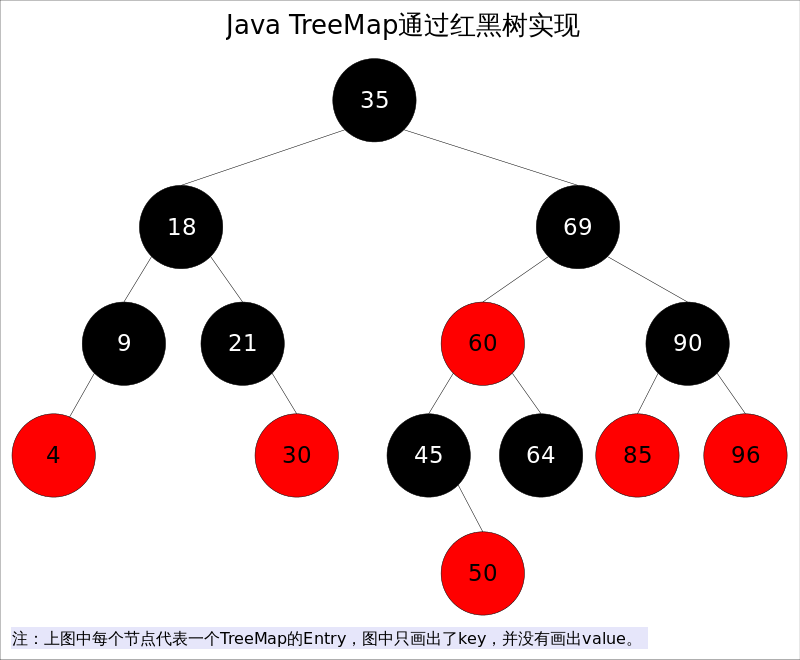SortedMap m = Collections.synchronizedSortedMap(new TreeMap(...));

1. 每个节点要么是红色，要么是黑色。
2. 根节点必须是黑色
3. 红色节点不能连续（也即是，红色节点的孩子和父亲都不能是红色）。
4. 对于每个节点，从该点至null（树尾端）的任何路径，都含有相同个数的黑色节点。

# 预备知识

## 左旋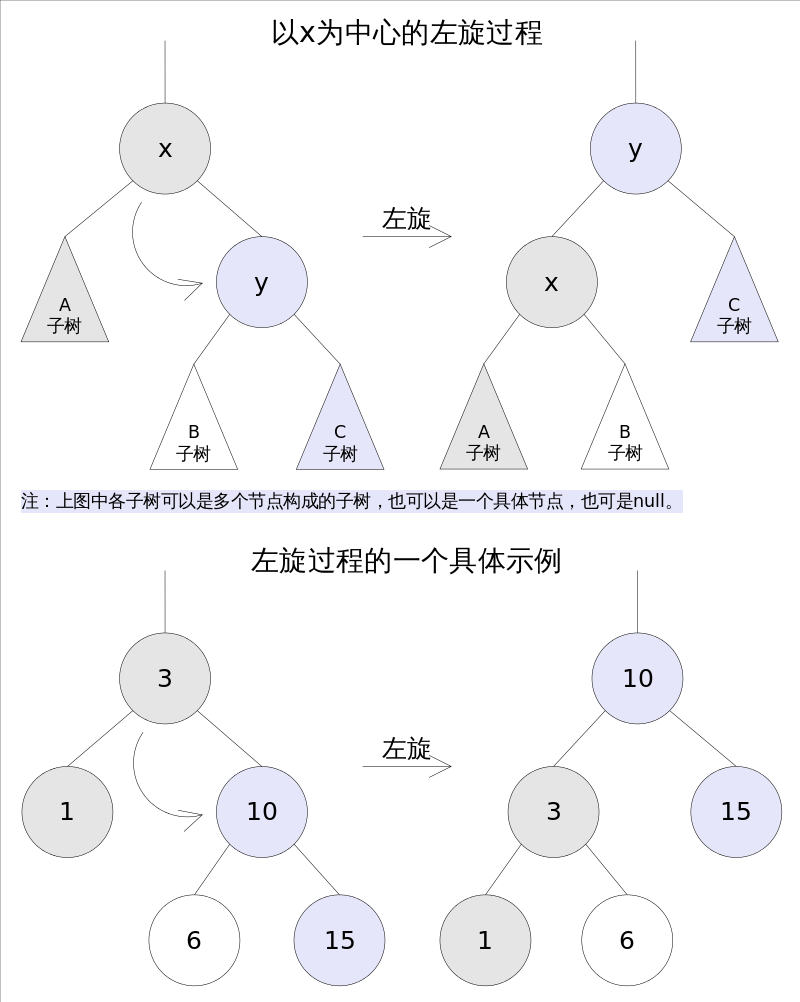TreeMap中左旋代码如下：

//Rotate Left
private void rotateLeft(Entry<K,V> p) {
if (p != null) {
Entry<K,V> r = p.right;
p.right = r.left;
if (r.left != null)
r.left.parent = p;
r.parent = p.parent;
if (p.parent == null)
root = r;
else if (p.parent.left == p)
p.parent.left = r;
else
p.parent.right = r;
r.left = p;
p.parent = r;
}
}

## 右旋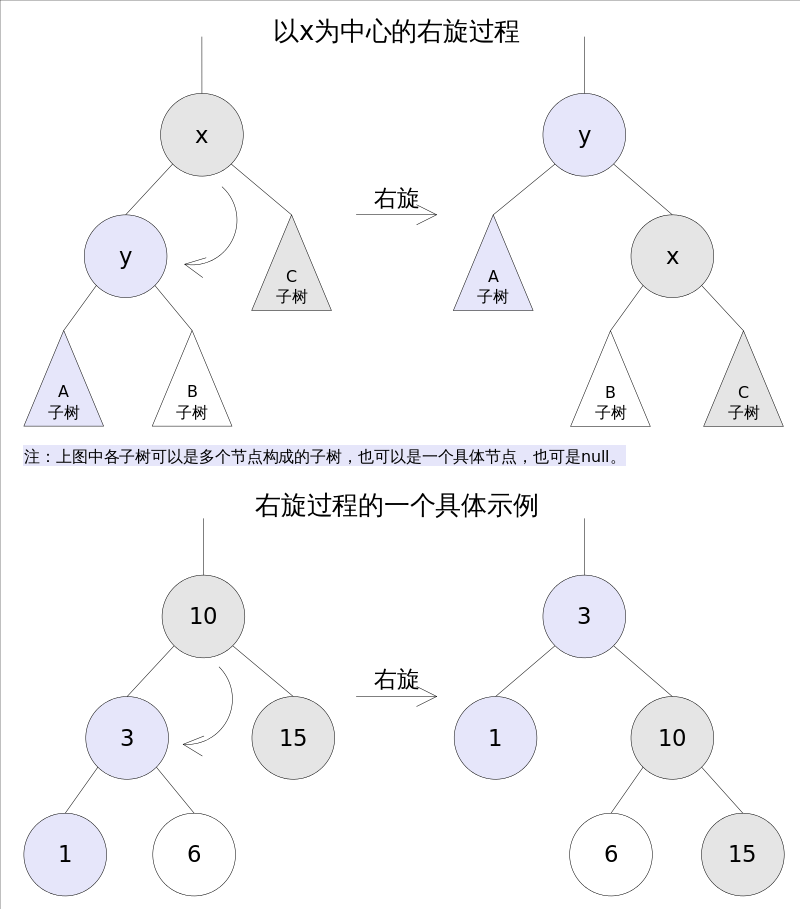TreeMap中右旋代码如下：

//Rotate Right
private void rotateRight(Entry<K,V> p) {
if (p != null) {
Entry<K,V> l = p.left;
p.left = l.right;
if (l.right != null) l.right.parent = p;
l.parent = p.parent;
if (p.parent == null)
root = l;
else if (p.parent.right == p)
p.parent.right = l;
else p.parent.left = l;
l.right = p;
p.parent = l;
}
}

# 方法剖析

## get()

get(Object key)方法根据指定的key值返回对应的value，该方法调用了getEntry(Object key)得到相应的entry，然后返回entry.value。因此getEntry()是算法的核心。算法思想是根据key的自然顺序（或者比较器顺序）对二叉查找树进行查找，直到找到满足k.compareTo(p.key) == 0entry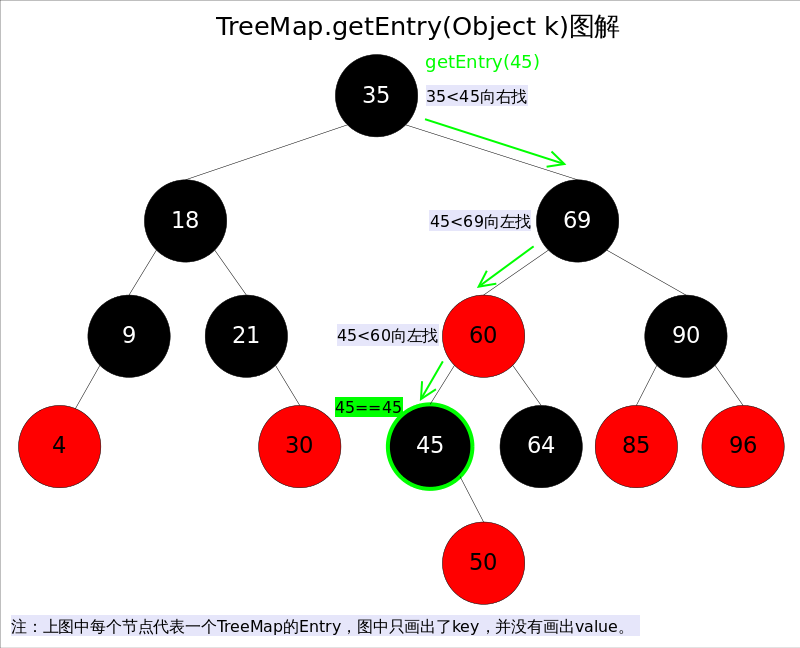//getEntry()方法
final Entry<K,V> getEntry(Object key) {
......
if (key == null)//不允许key值为null
throw new NullPointerException();
Comparable<? super K> k = (Comparable<? super K>) key;//使用元素的自然顺序
Entry<K,V> p = root;
while (p != null) {
int cmp = k.compareTo(p.key);
if (cmp < 0)//向左找
p = p.left;
else if (cmp > 0)//向右找
p = p.right;
else
return p;
}
return null;
}

## put()

put(K key, V value)方法是将指定的key, value对添加到map里。该方法首先会对map做一次查找，看是否包含该元组，如果已经包含则直接返回，查找过程类似于getEntry()方法；如果没有找到则会在红黑树中插入新的entry，如果插入之后破坏了红黑树的约束，还需要进行调整（旋转，改变某些节点的颜色）。

public V put(K key, V value) {
......
int cmp;
Entry<K,V> parent;
if (key == null)
throw new NullPointerException();
Comparable<? super K> k = (Comparable<? super K>) key;//使用元素的自然顺序
do {
parent = t;
cmp = k.compareTo(t.key);
if (cmp < 0) t = t.left;//向左找
else if (cmp > 0) t = t.right;//向右找
else return t.setValue(value);
} while (t != null);
Entry<K,V> e = new Entry<>(key, value, parent);//创建并插入新的entry
if (cmp < 0) parent.left = e;
else parent.right = e;
fixAfterInsertion(e);//调整
size++;
return null;
}

1.改变某些节点的颜色

2.对某些节点进行旋转。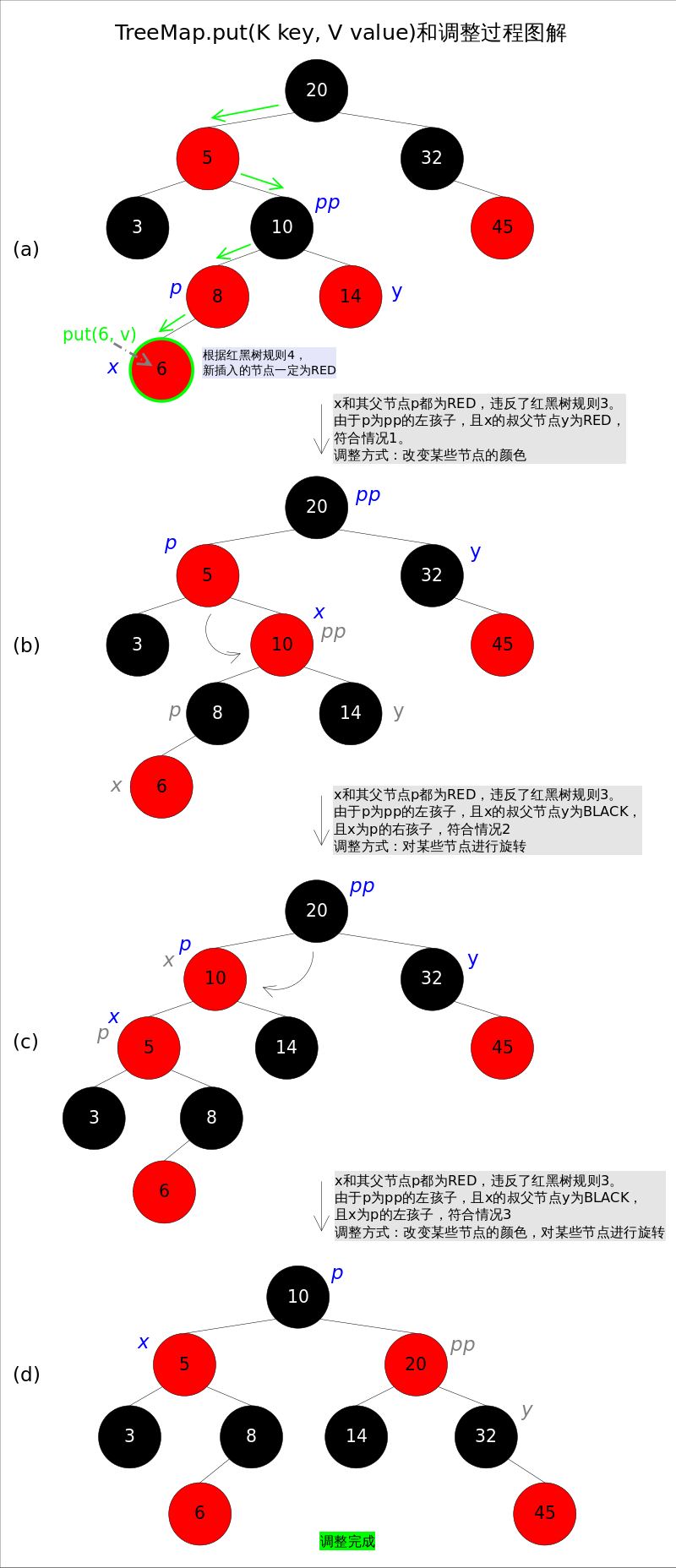//红黑树调整函数fixAfterInsertion()
private void fixAfterInsertion(Entry<K,V> x) {
x.color = RED;
while (x != null && x != root && x.parent.color == RED) {
if (parentOf(x) == leftOf(parentOf(parentOf(x)))) {
Entry<K,V> y = rightOf(parentOf(parentOf(x)));
if (colorOf(y) == RED) {//如果y为null，则视为BLACK
setColor(parentOf(x), BLACK);              // 情况1
setColor(y, BLACK);                        // 情况1
setColor(parentOf(parentOf(x)), RED);      // 情况1
x = parentOf(parentOf(x));                 // 情况1
} else {
if (x == rightOf(parentOf(x))) {
x = parentOf(x);                       // 情况2
rotateLeft(x);                         // 情况2
}
setColor(parentOf(x), BLACK);              // 情况3
setColor(parentOf(parentOf(x)), RED);      // 情况3
rotateRight(parentOf(parentOf(x)));        // 情况3
}
} else {
Entry<K,V> y = leftOf(parentOf(parentOf(x)));
if (colorOf(y) == RED) {
setColor(parentOf(x), BLACK);              // 情况4
setColor(y, BLACK);                        // 情况4
setColor(parentOf(parentOf(x)), RED);      // 情况4
x = parentOf(parentOf(x));                 // 情况4
} else {
if (x == leftOf(parentOf(x))) {
x = parentOf(x);                       // 情况5
rotateRight(x);                        // 情况5
}
setColor(parentOf(x), BLACK);              // 情况6
setColor(parentOf(parentOf(x)), RED);      // 情况6
rotateLeft(parentOf(parentOf(x)));         // 情况6
}
}
}
root.color = BLACK;
}

## remove()

remove(Object key)的作用是删除key值对应的entry，该方法首先通过上文中提到的getEntry(Object key)方法找到key值对应的entry，然后调用deleteEntry(Entry<K,V> entry)删除对应的entry。由于删除操作会改变红黑树的结构，有可能破坏红黑树的约束，因此有可能要进行调整。

# 告知

posted @ 2018-10-25 21:37 鄙人薛某 阅读(...) 评论(...) 编辑 收藏